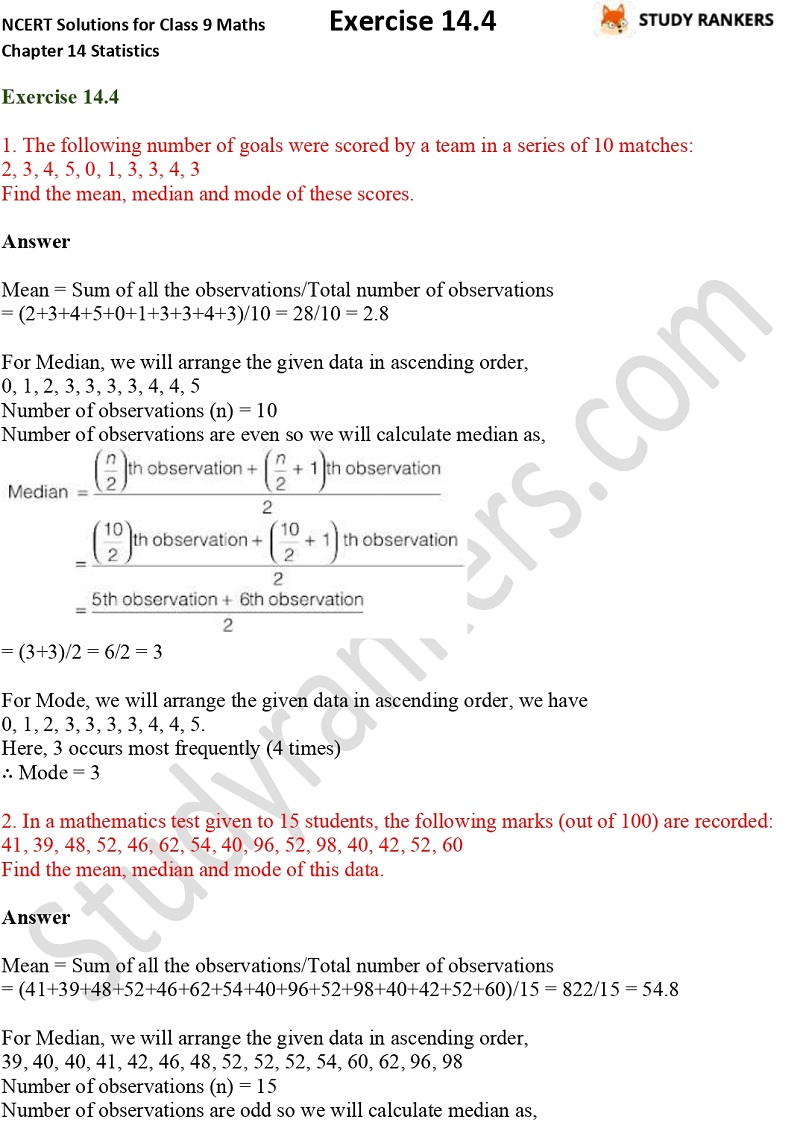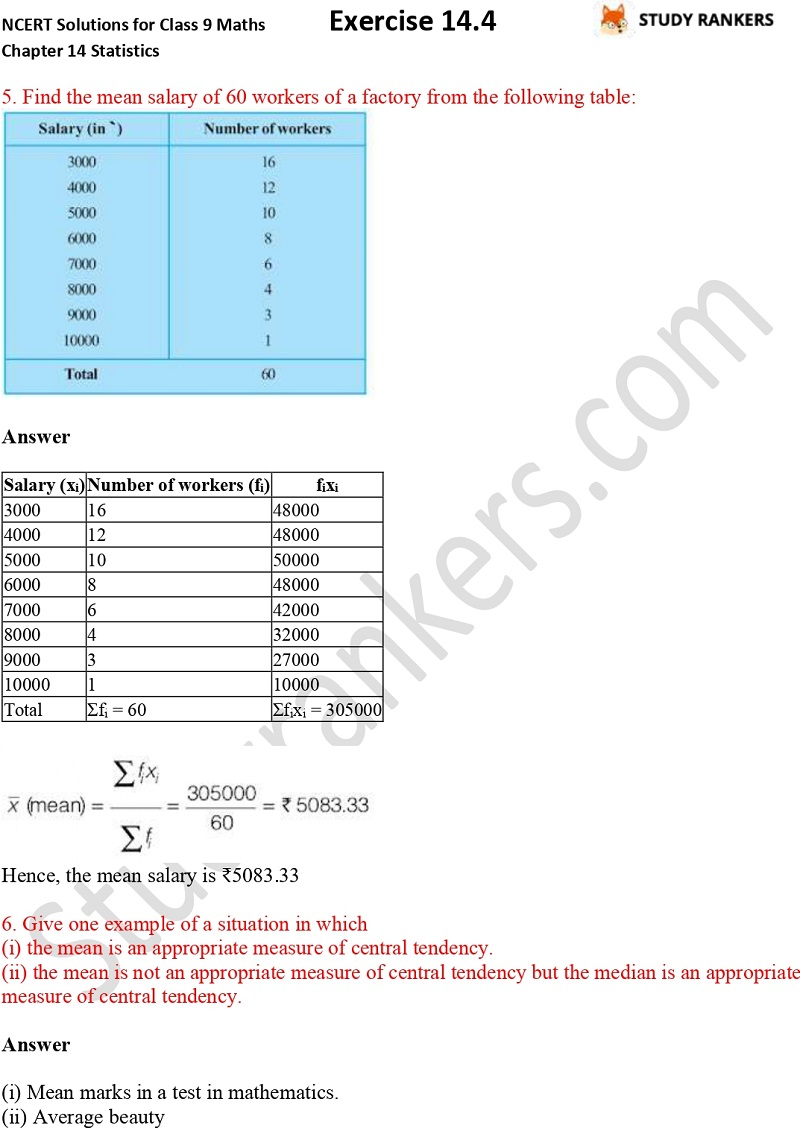>

## NCERT Solutions for Class 9 Maths Chapter 14 Statistics Exercise 14.4

You can find Chapter 14 Statistics Exercise 14.4 Maths Class 9 NCERT Solutions here that will be useful in knowing the basic concepts of the chapter. NCERT Solutions for Class 9 Maths is very helpful in completing your homework and improving your marks in the examination. The answers are prepared by Studyrankers subject matter experts that are detailed so you can understand the concepts easily. These NCERT Solutions are updated as per the latest syllabus of CBSE.

There are total six questions in the exercise 14.4 which are about finding the mean salary of the workers, Find the mean, median and mode of given data etc.X